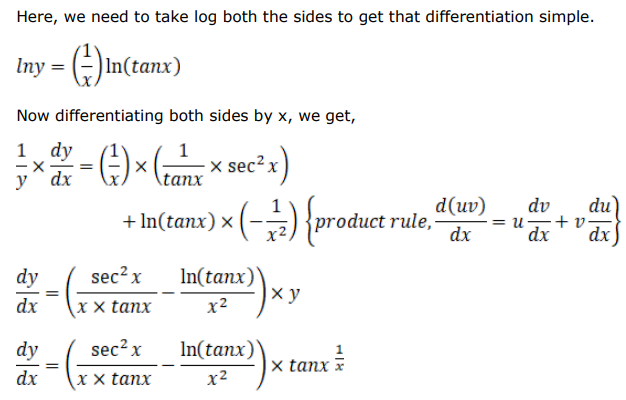# Solve this followingQuestion:

Find $\frac{d y}{d x}$, when:

$y=(\tan x)^{1 / x}$

Solution: